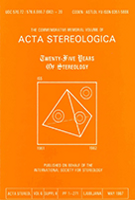Acta Stereologica -  Volume 10 (1991)  Number 1 - Stermat '90 (part I) - June 1991

## On some misunderstanding of fracture roughness parameters

Leszek Wojnar
Institute of Materials Science, Technical University, Al. Planu 6-letniego 19A, 31-864 Cracow, Poland

#### Abstract

A corrected equation describing the upper bound for RL—RS relation was derived as Rs = (π/2).RL – (π2 – 8)/4.

Due to some misunderstanding the above equation was called incorrect. The nature of this misunderstanding is explained and an experimental proof of correctness is presented.

Keywords : fractography, roughness parameters, stereometric equations, surface rougness